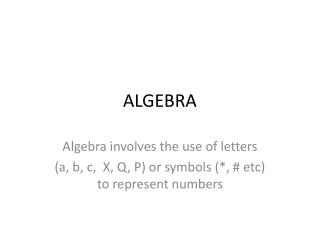DownloadDownload PresentationALGEBRA

# ALGEBRA

Télécharger la présentation## ALGEBRA

- - - - - - - - - - - - - - - - - - - - - - - - - - - E N D - - - - - - - - - - - - - - - - - - - - - - - - - - -
##### Presentation Transcript

1. ALGEBRA Algebra involves the use of letters (a, b, c, X, Q, P) or symbols (*, # etc) to represent numbers

2. Where is algebra used? • You would have seen algebra used in ‘formulae’ to work out things; • The area of a triangle A = ½ BH • Converting different currencies (money) • \$ AUS = 1.03 \$US

3. Some formulae involving algebra • Volume of a prism V = L x W x H • Area of a square A = L X L • Conversion of Km to miles K = 1.6M • Conversion of pound weight to Kg K = 2.2P

4. Why not use numbers? • Algebra is used where numbers can and will change. • In the previous example, the area of a triangle will change simply because triangles can have different heights and base measurements.

5. Algebra Rules (numbers and letters?) • What does 7h mean? • It means 7 ‘lots’ of h or more accurately 7 X h • (h + h + h + h + h + h + h) • When using algebra we assume that the operation of X is between numbers and letters

6. We don’t bother using ‘X’ • So 7h means 7 X h • 6hy means 6 X h X y • 34hjk means 34 X h X j X k

7. When using x as a letter • To avoid confusion between using the letter x and multiplication X it is best to use x. • For example 5xy means 5 x xx y and not confused with 5 x y

8. No need to use a single ‘1’ • We don’t bother writing 1g , 1w, 1p etc. Simply write g, w or p • fgh means f x g x h

9. What we can add or subtract. • To add or subtract algebraic terms the letters must be the same. • 6i + 7i = 13i • 6ui + 7i cannot be added!

10. Algebra (adding & subtracting terms) • We can add or subtract ‘terms’ as long as they contain the same letter or letters. (it is like collecting different fruits in separate bowls) • 6j - 5j = j • 8w + 4w = 12w • 5r - 2e (we cannot subtract because they have different letters)

11. Adding and subtracting algebra terms • 6t + 5a + 7t + 2a = • First look at the terms with ‘t’ we have 6t + 7t (that adds up to 13t) • Next look at the terms with ‘a’ we have 5a + 2a (that adds up to 7a) So the answer is 13t + 7a (we cannot add these together)

12. What about 7w - 7w = ? • 7w - 7w = 0w (anything multiplied by zero is zero) So answer is 0!

13. What about 4r - 5r = ? • Using our knowledge of directed numbers we know that 4 - 5 = - 1 • So 4r - 5r = -1r • Remember we don’t use ‘1’ so the answer can be written as - r

14. What about multiplication & divisionof algebraic terms? That’s a story for next time!

15. Have a go at these questions. • a + a + a = (e) 4t + 5r + 2t + 5r = • 3b + 2b = (f) 5f - 4f + 3g = • 3b + 2b + b = (g) 9j - 9j = • 5k - 2k - k = (h) 3e + 7r + 2r – 4e =

16. Practice questions • 6e + 6ei + 8e = • 10p -12p + 6p = • 100q -10q +12x = • 3a + 2b – a + 3b – b = • a – a + a – a + a + a = • 12y + 2x + 0 + 4y = • w - 10w + 2x – w = • 12xy+ 4x + 4y + 2xy = • a + b + a + b – 2a – b = • 7t + 7x + 7y + 7t + 2y = • p + q + r = • 12x– 2x– 7y – 9y =